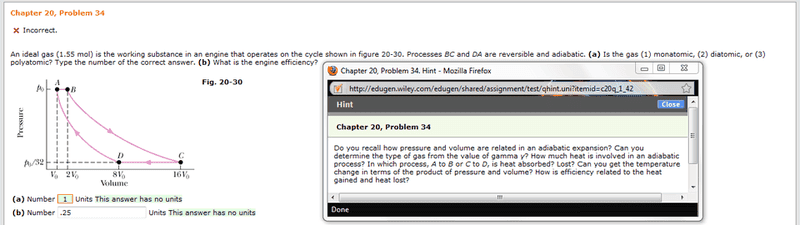# Physics - Calculating gamma

VitaX

## Homework Statementγ = Cp/Cv
ε = W/Qh
PiVi^γ = PfVf^γ

## The Attempt at a Solution

I know I have to find the gamma firstly in order to determine what type of gas is present here. And to do that I have to pick one of the adiabatic processes. I chose to do AD. But I am literally lost at how to set this up because we aren't really given values and reading graphs is not one of my strong suits in physics.

Note - The green in picture means "Correct". I just input 1 for the gas and .25 for the efficiency off guessing.

Homework Helper
γ = Cp/Cv
ε = W/Qh
PiVi^γ = PfVf^γ

## The Attempt at a Solution

I know I have to find the gamma firstly in order to determine what type of gas is present here. And to do that I have to pick one of the adiabatic processes. I chose to do AD. But I am literally lost at how to set this up because we aren't really given values and reading graphs is not one of my strong suits in physics.
To calculate the efficiency use:

ε = W/Qh = (Qh-Qc)/Qh

First, find the temperatures at A, B, C and D in terms of P0, and V0.

Then using the adiabatic condition expressed in terms of T and V, work out Cp/Cv. You will then be able to find Qh and Qc from the graph (for the constant pressure processes dQ = nCpdT) in terms of P0 and V0.

AM

Last edited:
VitaX
To calculate the efficiency use:

ε = W/Qh = (Qh-Qc)/Qh

First, find the temperatures at A, B, C and D in terms of P0, and V0.

Then using the adiabatic condition expressed in terms of T and V, work out Cp/Cv. You will then be able to find Qh and Qc from the graph (for the constant pressure processes dQ = nCpdT) in terms of P0 and V0.

AM
Do you mean using the ideal gas law PV = nRT?

Point A Temperature:
PoVo = 1.55*8.31*T
T = PoVo/12.8805

Point D Temperature:
(Po/32)*8Vo = 1.55*8.31*T
T = PoVo/51.522

Is this what you were saying?

Homework Helper
Do you mean using the ideal gas law PV = nRT?

Point A Temperature:
PoVo = 1.55*8.31*T
T = PoVo/12.8805

Point D Temperature:
(Po/32)*8Vo = 1.55*8.31*T
T = PoVo/51.522

Is this what you were saying?
Yes. Calculate T at B and C now using the same method. But show the relationships between them:

$$T_D = 8T_A/32 = T_A/4$$

AM